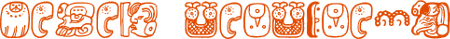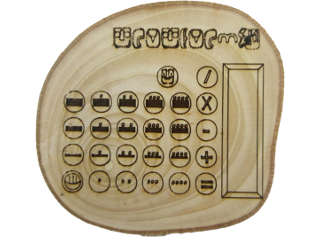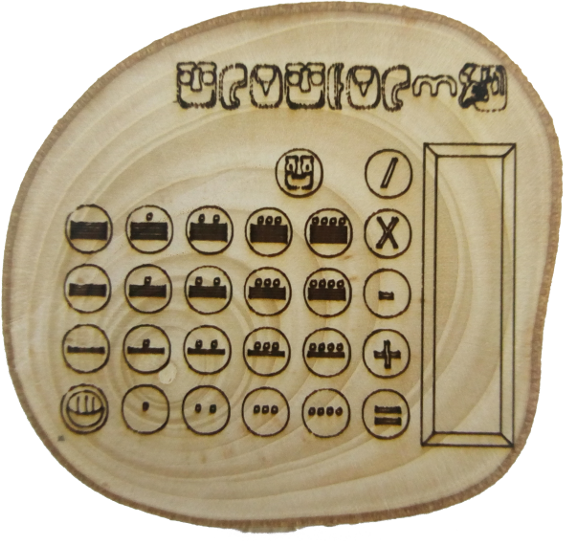###Mayan Calculator

2016#Mayan Calculator

I wanted to make a working Mayan calculator.

You can also view the calculator fullscreen

## Mayan Numbers

• Mayan numbers are base 20.
• Mayan digits are made of dots and lines. A dot is one and a line is 5; a bit like a tally.
• Mayan numbers are writtem vertically with the units at the bottom.

For example:### Mayan Numbers

Decimal         Mayan
 10,000 1,000 100 10 1
 160,000 8,000 400 20 1

## My Mayan Calculator

• The calculator only works with positive numbers. Any negative number will give a result of zero.
• It only works with whole numbers. Any decimals are rounded to a whole number.
• It only supports upto 6 Mayan digits which is upto 64,000,000 in decimal.

## What I Did

• First I designed the calculator on paper.### Wooden Calculator

• Then I made the calculator out of wood.
• I wanted the calculator to work so I took a picture of the wooden one I had made,
• I had to then make a font of the Mayan numbers. I did this on fontstruct.com  . Because there are 20 Mayan digits I used the capital letters A-T for the numbers. Mayan letters do not have capitals and lower case so I kept the lower case letter (a-z) for the Mayan letters. I only needed to make a Mayan "c" for the Clear button. I also added the basic maths symbols to my new Mayan font.
• I then needed to work out how to convert between base 20 and base 10 so I could do all the calculations in base 10.
• Finally, I wrote some Python code to make a working Mayan Calculator.